# Question about Ampere's Force Law derivation

• DirecSa
In summary, the conversation is about the derivation of Ampere's force law formula, which states that the effect of a current flowing in a circuit twisted into small sinuosities is the same as if the circuit were smoothed out. The article discussed in the conversation presents an equation (A1) derived using vector analysis. The article also discusses equation (A3) and why it is the simplest form. The derivation involves Maxwell's equations, Biot-Savart's law, and the Lorentz-force law. The force on a charge element in one loop is given by the integral of the magnetic field over the entire other loop.

#### DirecSa

I'm reading about the derivation of ampere force law formula, which is $$F=k_A \iint \frac{i'ds' \times (ids \times \vec{r})}{\vec{r}^2}$$ where K_A is mu_0/4pi. In the article that I read, they have assumed such these paths: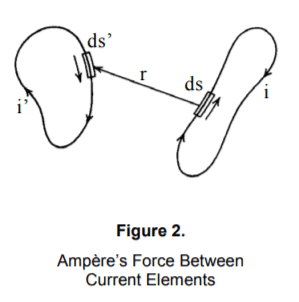And according to ampere's conclusion that he had from observation is that the effect of a current flowing in a circuit twisted into small sinuosities is the same as if the circuit were smoothed out. And then in the article they came up with the following equation (A1):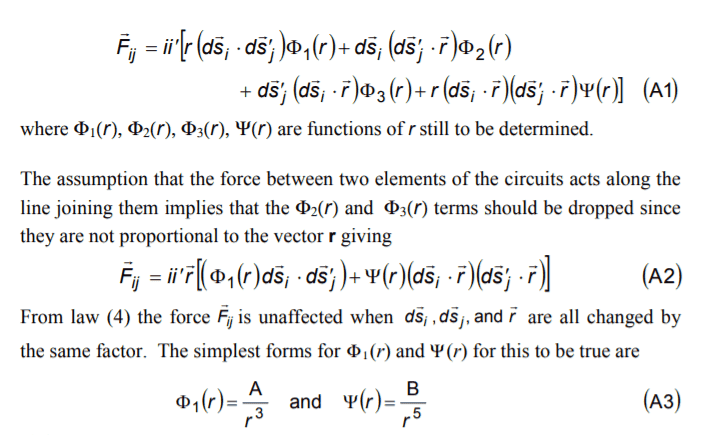So I guess that it came from vectors analysis, but I can't actually figure out how they got it...! In addition, my second question would be about equations (A3), why it would be the guess and why they are the simplest forms and not for example 1/r^2?
I would be grateful for explanations and thank you in advance.

The article is: Derivation of the Universal Force Law—Part 2 Charles W. Lucas, Jr. 29045 Livingston Drive Mechanicsville, MD 20659-3271

Last edited:
DirecSa said:
So I guess that it came from vectors analysis,
The text itself says otherwise: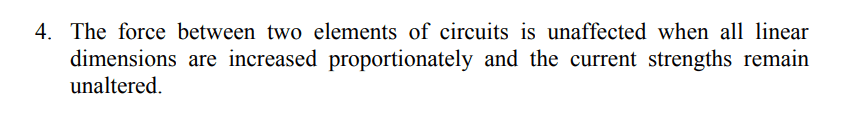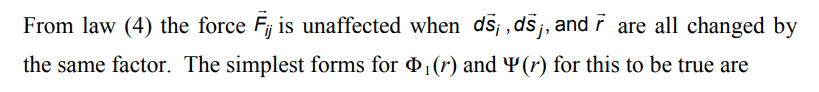... So it's just counting: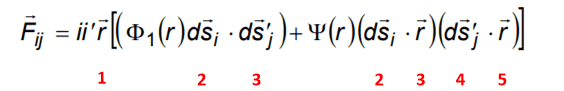And if e.g. ##\Phi_1\ ## would be proportional to ##\displaystyle{1\over r^2}\ ##, then ##F## would not stay the same if all ##r## and ##s## would be changed by a factor of e.g 2: F would double !

•DirecSa
It's very awful notation ;-). What's behind it is just Biot-Savart's Law for magnetostatic fields and the Lorentz-force law.

Let's start with the ##\vec{B}## field. The fundamental equations are Maxwell's equations,
$$\vec{\nabla} \cdot \vec{B}=0, \quad \vec{\nabla} \times \vec{B}=\mu_0 \vec{j}.$$
The first equation tells you that there's a vector potential ##\vec{A}## such that
$$\vec{B}=\vec{\nabla} \times \vec{A}.$$
Now ##\vec{A}## is determined only up to a gradient of an arbitrary scalar field (gauge invariance), and thus you can impose one "gauge constraint", which in this case is best chosen as the Coulomb-gauge condition,
$$\vec{\nabla} \cdot \vec{A}=0.$$
Then plug this into the 2nd equation:
$$\vec{\nabla} \times \vec{B}=\vec{\nabla} \times (\vec{\nabla} \times \vec{A}) = \vec{\nabla} (\vec{\nabla} \cdot \vec{A})-\Delta \vec{A}=-\Delta \vec{A}=\mu_0 \vec{j}.$$
From electrostatics ("Coulomb's Law") you know how to solve that equation:
$$\vec{A}(\vec{x})=\int_{\mathbb{R}} \mathrm{d}^3 x' \frac{\mu_0 \vec{j}(\vec{x}')}{4 \pi |\vec{x}-\vec{x}'|}.$$
Taking the curl leads to Biot-Savart's law for arbitrary current distributions
$$\vec{B}=\vec{\nabla} \times \vec{A}=\frac{\mu_0}{4 \pi} \int_{\mathbb{R}} \mathrm{d}^3 x' \vec{j}(\vec{x}') \times \frac{\vec{x}-\vec{x}'}{|\vec{x}-\vec{x}'|^3}.$$
If you now idealize your loop as an infinitely thin wire you have (by choosing the appropriate sign for the direction of the path)
$$\mathrm{d}^3 x' \vec{j}(\vec{x}')=\mathrm{d} \vec{x}' i,$$
$$\vec{B}(\vec{x})=\frac{\mu_0 i}{4 \pi} \int_{C} \mathrm{d} \vec{x}' \times \frac{\vec{x}-\vec{x}'}{|\vec{x}-\vec{x}'|^3}.$$
Now the force on a little charge element in the other loop is
$$\mathrm{d} \vec{F}=\mathrm{d} q \vec{v} \times \vec{B}=i \mathrm{d} \vec{r} \times \vec{B},$$
from which you get the claimed result integrating over the entire loop ##C'##
$$\vec{F}=\frac{\mu_0}{4 \pi} \int_{C'} \mathrm{d} \vec{x} \times \int_{C} \mathrm{d} \vec{x}' \times \frac{\vec{x}-\vec{x}'}{|\vec{x}-\vec{x}'|^3}.$$

•DirecSa and Delta2
vanhees71 said:
It's very awful notation ;-). What's behind it is just Biot-Savart's Law for magnetostatic fields and the Lorentz-force law.

Let's start with the ##\vec{B}## field. The fundamental equations are Maxwell's equations,
$$\vec{\nabla} \cdot \vec{B}=0, \quad \vec{\nabla} \times \vec{B}=\mu_0 \vec{j}.$$
The first equation tells you that there's a vector potential ##\vec{A}## such that
$$\vec{B}=\vec{\nabla} \times \vec{A}.$$
Now ##\vec{A}## is determined only up to a gradient of an arbitrary scalar field (gauge invariance), and thus you can impose one "gauge constraint", which in this case is best chosen as the Coulomb-gauge condition,
$$\vec{\nabla} \cdot \vec{A}=0.$$
Then plug this into the 2nd equation:
$$\vec{\nabla} \times \vec{B}=\vec{\nabla} \times (\vec{\nabla} \times \vec{A}) = \vec{\nabla} (\vec{\nabla} \cdot \vec{A})-\Delta \vec{A}=-\Delta \vec{A}=\mu_0 \vec{j}.$$
From electrostatics ("Coulomb's Law") you know how to solve that equation:
$$\vec{A}(\vec{x})=\int_{\mathbb{R}} \mathrm{d}^3 x' \frac{\mu_0 \vec{j}(\vec{x}')}{4 \pi |\vec{x}-\vec{x}'|}.$$
Taking the curl leads to Biot-Savart's law for arbitrary current distributions
$$\vec{B}=\vec{\nabla} \times \vec{A}=\frac{\mu_0}{4 \pi} \int_{\mathbb{R}} \mathrm{d}^3 x' \vec{j}(\vec{x}') \times \frac{\vec{x}-\vec{x}'}{|\vec{x}-\vec{x}'|^3}.$$
If you now idealize your loop as an infinitely thin wire you have (by choosing the appropriate sign for the direction of the path)
$$\mathrm{d}^3 x' \vec{j}(\vec{x}')=\mathrm{d} \vec{x}' i,$$
$$\vec{B}(\vec{x})=\frac{\mu_0 i}{4 \pi} \int_{C} \mathrm{d} \vec{x}' \times \frac{\vec{x}-\vec{x}'}{|\vec{x}-\vec{x}'|^3}.$$
Now the force on a little charge element in the other loop is
$$\mathrm{d} \vec{F}=\mathrm{d} q \vec{v} \times \vec{B}=i \mathrm{d} \vec{r} \times \vec{B},$$
from which you get the claimed result integrating over the entire loop ##C'##
$$\vec{F}=\frac{\mu_0}{4 \pi} \int_{C'} \mathrm{d} \vec{x} \times \int_{C} \mathrm{d} \vec{x}' \times \frac{\vec{x}-\vec{x}'}{|\vec{x}-\vec{x}'|^3}.$$

Thank you so much for this approach, that's really helpful :)

•vanhees71
BvU said:
The text itself says otherwise:

View attachment 278726
View attachment 278725
... So it's just counting:

View attachment 278727

And if e.g. ##\Phi_1\ ## would be proportional to ##\displaystyle{1\over r^2}\ ##, then ##F## would not stay the same if all ##r## and ##s## would be changed by a factor of e.g 2: F would double !

Thank you a lot for your comment. Actually I didn't understand what do you mean about counting...? And how did you deduce that from the law number 4 and what does that mean?
Regarding function ##\Phi##, how it stay the same with power 3 (or power 5)?!

DirecSa said:
Thank you a lot for your comment. Actually I didn't understand what do you mean about counting...? And how did you deduce that from the law number 4 and what does that mean?
Regarding function ##\Phi##, how it stay the same with power 3 (or power 5)?!
You count the places where ##d\vec s## and ##\vec r## occur. The ##\vec r## in front of the [ bracket is common and ##\Phi## is followed by ##d\vec s_i\cdot d\vec s_j##. So if all are changed by factor of 2, ##\vec F_{ij}## can only stay the same if ##\Phi## is changed by a factor of 1/8 .
For ##\Psi## 1/32.

##\ ##

•DirecSa
BvU said:
You count the places where and occur. The in front of the [ bracket is common and is followed by . So if all are changed by factor of 2, can only stay the same if is changed by a factor of 1/8 .
For 1/32.

Maybe now I have some direction to think about it, thank you :)

DirecSa said:
I would like to know how they wrote equation A1, I tried to understand how they came up to this equation but I couldn't figure out how. Please if you can give me the mathematical concept or the logic behind it and that they used, and why should be like this I have no clue at all.
Better to ask in the forum -- that way others can correct mistakes and genuine nonsense !

We have a problem in the sense that we are discussing stuff from a source that PF doesn't approve of.
commonsensescience.org is now .net (*)

See this link under Acceptable sources.

I responded to your original post because the answer was fairly straightforward. And I personally don't mind to help with plowing through this guy's stuff, but I think you'd be better off working through e.g. Jackson ...

Lucas said:
Ampère  performed a series of four experiments and found that the force between two current elements in current loops obey the following laws:
1. The effect of a current is reversed when the direction of the current is reversed.
2. The effect of a current flowing in a circuit twisted into small sinuosities is the same as if the circuit were smoothed out.
3. The force exerted by a closed circuit on an element of another circuit is at right angles to the latter.
4. The force between two elements of circuits is unaffected when all linear dimensions are increased proportionately and the current strengths remain unaltered.
From law (2) the effect of ds on ds’ is the vector sum of the effects on ds’. From law (1) which supports Newton’s third law of action and reaction plus the assumption that the force is linear and homogeneous in ds and ds’, the simplest general formula must be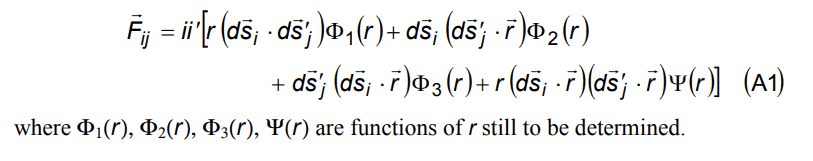Isn't that just the sum of what is left over of the possible varieties ?

##\ ##

(*) Look where this guy wants to take you ! :
their website said:
Dr. Charles W. (Bill) Lucas
Dr. Charles Lucas earned his doctorate in Intermediate Energy Physics from The College of William and Mary in Williamsburg, Virginia in 1972 and then performed post-graduate research on pions at Catholic University in Washington, DC. He has derived a universal electro-dynamic force law that describes physical phenomena on all size scales throughout the universe including what he terms "the pulse of life," on the molecular scale and a new paradigm for medicine. Using the universal force law he has refined the ring model to describe the complete set of elementary particles, their decay schemes, reactions, and excited states by introducing the classical concept of stable elementary particles being composed of primary, secondary and tertiary level three dimensional closed strings that reflect the chiral symmetry of the universal force. This classical model conserves energy, total number of charge strings, and unique angular momentum configurations of the primary strings in all decays and reactions. Bill is a frequent speaker at science conferences and has been featured in many programs by Cornerstone TV on a syndicated program titled "Origins." This past year (2007) Bill presented a three credit 45 hour seminary course on creation at the International Baptist Bible College of Ukraine in Russian based on the universal force being identified from the Bible as the Divine force. From the universal force alone Bill was able to describe many aspects of the creation, the mechanism causing Noah's flood, what happened to all the water after the flood, and what caused the division of the continents 101 years later.

 ​

•DirecSa
BvU said:
Better to ask in the forum -- that way others can correct mistakes and genuine nonsense !

We have a problem in the sense that we are discussing stuff from a source that PF doesn't approve of.
commonsensescience.org is now .net (*)

See this link under Acceptable sources.

I responded to your original post because the answer was fairly straightforward. And I personally don't mind to help with plowing through this guy's stuff, but I think you'd be better off working through e.g. Jackson ...

Isn't that just the sum of what is left over of the possible varieties ?

##\ ##

(*) Look where this guy wants to take you ! :

 ​

I got your point, I didn't know that before, thank you a lot :)

•berkeman
It seems that the OP has enough help to continue his research and so it’s time to close this thread before someone new diverts us into fringe science.

Thanks to @DirecSa for asking this question and to @BvU for answering and discovering the fringe science aspect.

•DirecSa and BvU

## 1. What is Ampere's Force Law?

Ampere's Force Law is a mathematical equation that describes the force between two parallel current-carrying wires. It states that the force per unit length between two parallel conductors is directly proportional to the current in each conductor and inversely proportional to the distance between them.

## 2. How is Ampere's Force Law derived?

Ampere's Force Law is derived from the combination of two fundamental laws of electromagnetism: Ampere's Law and the Lorentz Force Law. By applying these laws to a simple system of two parallel current-carrying wires, the equation for the force between them can be derived.

## 3. What are the assumptions made in Ampere's Force Law derivation?

There are a few key assumptions made in the derivation of Ampere's Force Law. These include: the wires are infinitely long, straight, and parallel; the current is constant along the length of the wires; and the magnetic fields created by the wires are uniform.

## 4. How is Ampere's Force Law used in practical applications?

Ampere's Force Law is used in various practical applications, such as in the design of electromagnets and electric motors. It is also used in industries like telecommunications and power generation to calculate the force between power lines and to ensure safe spacing between them.

## 5. Are there any limitations to Ampere's Force Law?

Yes, there are some limitations to Ampere's Force Law. It is only applicable to parallel current-carrying wires and cannot be used for other configurations. Additionally, it does not take into account the effects of magnetic materials or nonlinear magnetic fields.